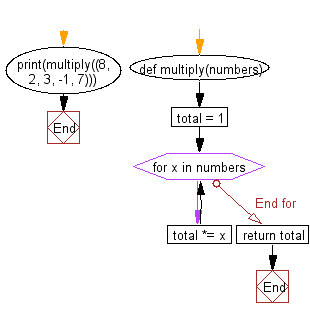﻿ Python Exercise: Multiply all the numbers in a list - w3resource# Python Exercise: Multiply all the numbers in a list

## Python Functions: Exercise-3 with Solution

Write a Python function to multiply all the numbers in a list.

Sample Solution:-

Python Code:

``````def multiply(numbers):
total = 1
for x in numbers:
total *= x
print(multiply((8, 2, 3, -1, 7)))
```
```

Sample Output:

```-336
```

Pictorial presentation:Flowchart:## Visualize Python code execution:

The following tool visualize what the computer is doing step-by-step as it executes the said program:

Python Code Editor:

Have another way to solve this solution? Contribute your code (and comments) through Disqus.

What is the difficulty level of this exercise?

Test your Python skills with w3resource's quiz

﻿

## Python: Tips of the Day

Creates a dictionary with the same keys as the provided dictionary and values generated by running the provided function for each value

Example:

```def tips_map_values(obj, fn):
ret = {}
for key in obj.keys():
ret[key] = fn(obj[key])
return ret
users = {
'Owen': { 'user': 'Owen', 'age': 29 },
'Eddie': { 'user': 'Eddie', 'age': 15 }
}

print(tips_map_values(users, lambda u : u['age'])) # {'Owen': 29, 'Eddie': 15}
```

Output:

```{'Owen': 29, 'Eddie': 15}
```